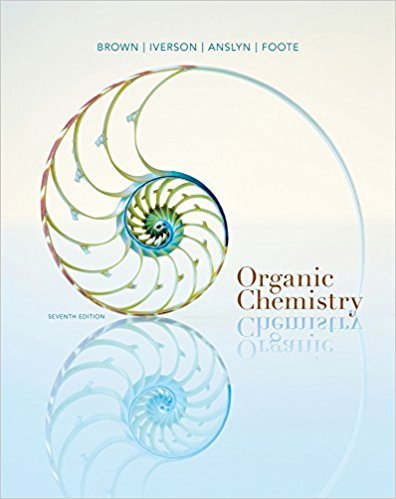×
Get Full Access to Organic Chemistry - 7 Edition - Chapter 21 - Problem 21.33
Get Full Access to Organic Chemistry - 7 Edition - Chapter 21 - Problem 21.33

×

# Account for the fact that water-insoluble carboxylic acids (pKa 45) dissolve inISBN: 9781133952848 483

## Solution for problem 21.33 Chapter 21

Organic Chemistry | 7th Edition

• Textbook Solutions
• 2901 Step-by-step solutions solved by professors and subject experts
• Get 24/7 help from StudySoup virtual teaching assistantsOrganic Chemistry | 7th Edition

4 5 1 298 Reviews
13
2
Problem 21.33

Account for the fact that water-insoluble carboxylic acids (pKa 45) dissolve in 10%aqueous sodium bicarbonate (pH 8.5) with the evolution of a gas, but that water-insoluble phenols (pKa 9.510.5) do not dissolve in 10% sodium bicarbonate.

Step-by-Step Solution:
Step 1 of 3

Devan Atteberry 4/14/2016 Chem 312 Week #3: Saturated/Unsaturated Hydrocarbons and Pi Bonds Major Structure at pH - Equilibrium A+ B= C+D o Equilibrium expression is Keq  Keq= (c) (d)/ (a) (b) or products/reactants  Keq=0 when no products are created  Keq=1 when products and reactants are the same in amount - pH o pH 7 is close to the biological conditions within human body - To do the structure and pH questions: o First find the most acidic Hydrogen on the molecule presented  Look for functional groups  Carboxylic acids generally 4.8 pKa  Generic C-H bonds generally 49 pKa  Alcohols are generally 17 pKa o If pH is less than pKa, solution isn’t basic enough to remove acidic hydrogen  pKa values higher than pH, hydrogen remain  pKa values lower than pH, hydrogen is removed o This entire process is called pronating Saturated Hyrdocarbons - Saturated means no rings or pi bonds contained in the molecule presented - General formal is Cn H2n+2 - 1 carbon (CH4)- methane - 2 carbon (H3CCH2) - ethane - 3 carbon (H3CCH2CH3)- propane - 4 ca

Step 2 of 3

Step 3 of 3

##### ISBN: 9781133952848

Organic Chemistry was written by and is associated to the ISBN: 9781133952848. This textbook survival guide was created for the textbook: Organic Chemistry, edition: 7. Since the solution to 21.33 from 21 chapter was answered, more than 252 students have viewed the full step-by-step answer. The answer to “Account for the fact that water-insoluble carboxylic acids (pKa 45) dissolve in 10%aqueous sodium bicarbonate (pH 8.5) with the evolution of a gas, but that water-insoluble phenols (pKa 9.510.5) do not dissolve in 10% sodium bicarbonate.” is broken down into a number of easy to follow steps, and 36 words. The full step-by-step solution to problem: 21.33 from chapter: 21 was answered by , our top Chemistry solution expert on 03/16/18, 05:02PM. This full solution covers the following key subjects: . This expansive textbook survival guide covers 24 chapters, and 1131 solutions.

## Discover and learn what students are asking

Calculus: Early Transcendental Functions : Differential Equations: Separation of Variables
?In Exercises 1-14, find the general solution of the differential equation. $$x^{2}+5 y \frac{d y}{d x}=0$$

Statistics: Informed Decisions Using Data : The Normal Probability Distribution
?Find the z-scores that separate the middle 92% of the data from the area in the tails of the standard normal distribution.

Unlock Textbook Solution